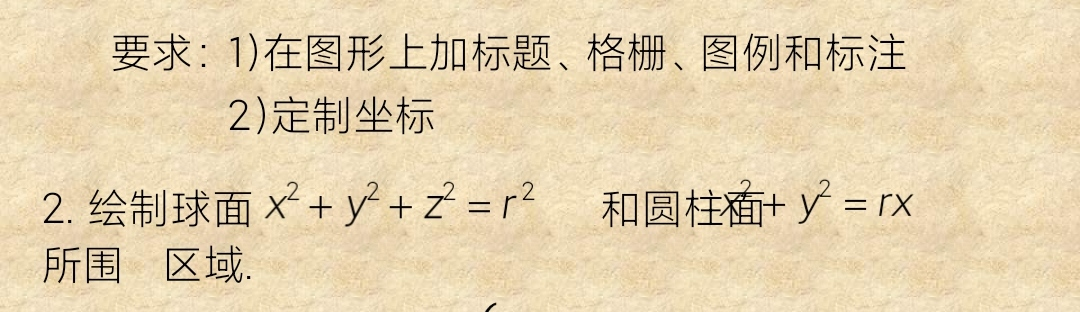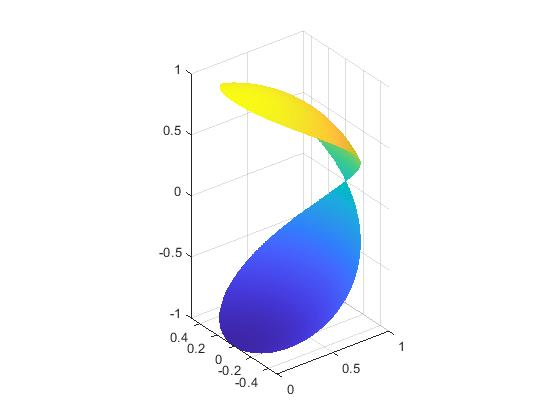qq_68017017 2022-03-12 18:57 采纳率: 100%

# 编程不会写求指导，该怎么用matlab来写• 写回答

#### 1条回答默认 最新

•joel_1993 2022-03-12 20:11
关注

你好可以这样做

``````
r = 1;
theta = linspace(0, 2*pi, 1001);
phi = linspace(0, pi, 1001);
[T, P] = meshgrid(theta, phi);
x = r*cos(T).*sin(P);
y = r*sin(T).*sin(P);
z = r*cos(P);
p = x.^2+y.^2>r*x;
x(p) = nan;
y(p) = nan;
z(p) = nan;
mesh(x,y,z)
axis equal

``````

效果本回答被题主选为最佳回答 , 对您是否有帮助呢?
评论

#### 悬赏问题

• ¥15 询问MYSQL查询SQLSERVER数据表并比较差异后，更新MYSQL的数据表
• ¥15 关于#前端#的问题，请各位专家解答！
• ¥15 最小生成树问题 Prim算法和Kruskal算法
• ¥25 医院住院病人呼叫器设计
• ¥15 不想和现在的团队合作了，怎么避免他们对程序动手脚
• ¥20 C语言字符串不区分大小写字典排序相关问题
• ¥15 关于#python#的问题：我希望通过逆向技术爬取1688搜索页下滑加载的数据
• ¥15 关于Linux的终端里，模拟实现一个带口令保护的屏保程序遇到的输入输出的问题！(语言-c语言)
• ¥30 请问，这个嵌入式Linux系统谁能帮我分析一下，crc检验区域在哪，不是内核的校验，内核校验我已经找到了
• ¥15 二分类改为多分类问题